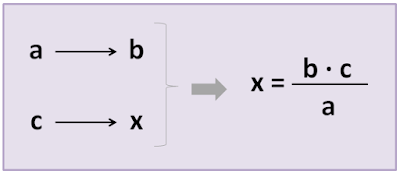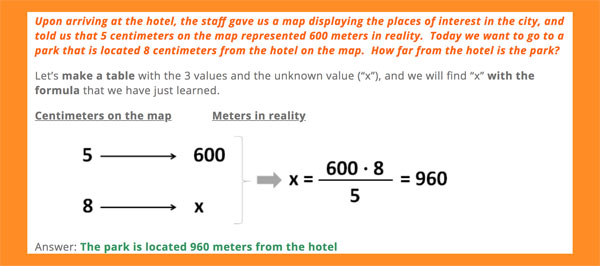## Monday, 4 February 2019

### Unit 6 - Rule of three

Hello, hello!
Now that we know about direct proportionality, we're learning the rule of three in Mathematics.The rule of three is an operation that helps us quickly solve both direct proportion word problems. In order to use the rule of three, we need three values; two that are proportional to one another, and a third. From there, we will figure out the fourth value.

### Direct rule of three

We will place the 3 values (which we will call “a”, “b”, and “c”) and the unknown value that we want to figure out (“x”) in a table. Next, we will apply the following formula:Direct rule of threeExamples of rule of three

### Exercises on the rule of three:

#### 3 comments:

1.i have done the worksheet.
patri

2.Im studing maths🤓🤓.See you tonorow😘💕

Martina M

3.This worksheet helps me a lot
Marisa=)